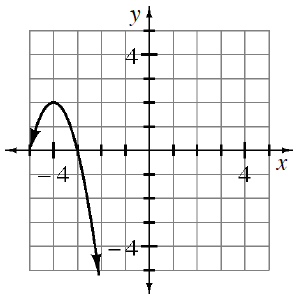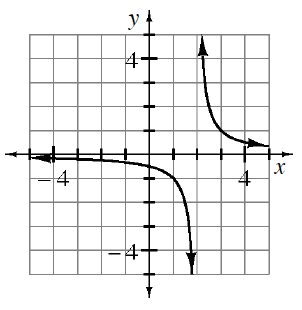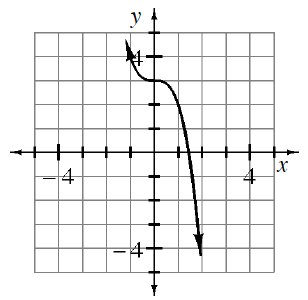### Home > INT3 > Chapter 3 > Lesson 3.1.1 > Problem3-15

3-15.

Write a possible equation for each graph.

1.What kind of graph is this?

Where is the vertex and what is another point on the graph?

Use the vertex to write an equation in graphing form.
Then substitute the coordinates of another point in for $x$ and $y$ to solve for $a$.
$y=a(x+4)^2+2$

1.The parent graph is $y=\frac{1}{x}$and the locator point is $\left(2,\ 0\right)$.

1.This is a cubic function that is decreasing instead of increasing.